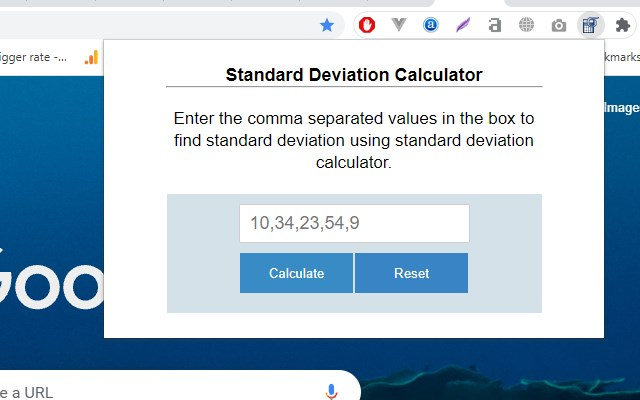# Standard Deviation Calculator

## This free standard deviation calculator computes the standard deviation, variance, mean, sum, and error margin of a given data set.###### What is Standard Deviation Calculator?

Standard Deviation Calculator is a free Edge add-on that computes the standard deviation, variance, mean, sum, and error margin of a given data set.##### Stats
Stats date:View on Edge Add-on Store
Users: 80
Version: 0.1.0 (Last updated: 2021-11-03)
Creation date: 2021-11-03
Manifest version: 2
Permissions:
• https://www.standarddeviationcalculator.io/inc/calculations.php
Size: 56.02K
URLs:
Risk impact: Low risk impact
Risk likelihood: Low risk likelihood

### Other platformsStandard Deviation Calculator (v0.1.0)
0.00 (0) 11Not available on Firefox##### Summary

This free standard deviation calculator computes the standard deviation, variance, mean, sum, and error margin of a given data set.

##### Safety
###### Risk impact

Standard Deviation Calculator is relatively safe to use as it requires very minimum permissions.

###### Risk likelihood

Standard Deviation Calculator has earned a fairly good reputation and likely can be trusted.